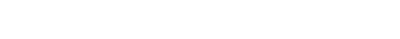# Introduction to Three Dimensional GeometryChapter 12

Introduction to Three Dimensional Geometryx- axis : (x,0,0)

y- axis : (0,y,0)

z- axis : (0,0,z)

XY-plane : (x,y,0)

YZ-plane : (0,y,z)

ZX-plane : (x,0,z)

+ + + : I

– + + : II

– –  + : III

+ – + :  IV

+ + – : V

– + – : VI

– – – : VII

+ – –  : VIII

Q. (4,-2,3)

IV   XOYIZ

Q. (-4,2,5)

II   XIOYZ

The distance of the point (a,b,c) from x-axisThe distance of the point (a,b,c) from y-axisThe distance of the point (a,b,c) from z-axisQ. Find the distance of  the point (2,3,4) from y-axisThe image of (a,b,c) w.r.to XY-plane  : (a,b,-c)

The image of (a,b,c) w.r.to YZ-plane  : (-a,b,c)

The image of (a,b,c) w.r.to ZX-plane  : (a,-b,c)

Q. Find the image of (2,-3,-4) w.r.to XY-plane

(2,-3,4)

Distance formulaQ. Using distance formula show that the points P(-2,3,5), Q(1,2,3) and R(7,0,-1) are collinearPoints are collinear

Q. Find the equation of set of points P which are equidistant from A(1,2,3) and B(3,2,-1)

Let the coordinates of the point P be (x,y,z)Section Formula

Internal divisionExternal divisionMidpoint =Q. Find the ratio in which the line segment joining (4,8,10) and (6,10,-8) is divided by YZ- plane

Let k:1 be the ratio

x coordinate of a point in YZ-plane is 0k.6+ 1.4 = 0

6k+4 = 0Externally in the ration 2 : 3

Q. Find the coordinates of a point which trisect the line segment joining (4,2,-6) and

(10,-16,6)

A divides the line segment in the ratio 1:2B divides the line segment in the ratio 2:1Q. Using section formula prove that the three points A(-4,6,10), B(14,0,-2) and C(2,4,6) are collinear

Assume that B divides AC in the ratio k:12k-4 = 14(k+1)

2k-4 = 14k + 14

-12k = 18

K = –  3/2

B divides AC in the ratio 3:2 externally

The points are collinear

Centroid of a triangle

The meeting point of three medians of a triangle is called centroid. Centroid divides each median in thr ratio 2:1

Centroid =Q. If the origin is the centroid of a triangle with vertices (2a,2,6), (-4,3b,-10) and (8,14,2c) find the values of a,b and c

Centroid2a+4 = 0

a = -23b+16 = 0

b = – 16/3

(2c-4)/3 = 0

2c – 4 = 0

c = 2